# Electronic Dispersion Compensation

Although electronic dispersion compensation attracted attention as early as 1990 because of its potential low cost and ease of implementation in the form of a integrated-circuit chip within the receiver, it was only after 2000 that it advanced enough to become usable in real lightwave systems. The main limitation of electronic techniques is related to the speed of electronic circuits. Recent advances in digital signal processing (DSP) have made electronic compensation a practical tool not only for GVD but also for PMD.

#### 1. Basic Idea Behind GVD Precompensation

The philosophy behind electronic techniques for GVD compensation is that, even though the optical signal has been degraded by GVD, one should be able to equalize the effects of dispersion electronically if the fiber acts as a linear system. When the GVD effects dominates, the transfer function of a fiber link of length L can be written aswhere da is the dispersion accumulated along the entire fiber link. If the electric signal generated at the receiver recovers both the amplitude and phase of the optical signal, one may be able to compensate for GVD by passing it through a suitable electrical filter. Unfortunately, the use of direct detection recovers only the amplitude, making it impossible to apply such a filter.

The situation is different in the case of coherent detection. It is relatively easy to compensate for dispersion if a heterodyne receiver is used for signal detection. Such a receiver first converts the optical signal into a microwave signal at the intermediate frequency ωIF, while preserving both the amplitude and phase information. A microwave bandpass filter whose impulse response is governed by the transfer function,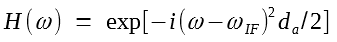restores the signal to is original form. Indeed, as early as 1992, a 31.5-cm-long microstrip line was used for dispersion equalization. Its use made it possible to transmit an 8-Gb/s signal over 188 km of standard fiber. In a 1993 experiment, the technique was extended to homodyne detection, and a 6-Gb/s signal could be recovered at the receiver after propagating over 270 km of standard fiber. Microstrip lines can be designed to compensate for GVD acquired over fiber lengths as long as 4,900 km for a lightwave system operating at a bit rate of 2.5 Gb/s.

In the case of direct-detection receivers, no linear equalization technique based on optical filters can recover a signal that has spread outside its allocated bit slot. Nevertheless, several nonlinear equalization techniques have been developed that permit recovery of the degraded signal. In one method, the decision threshold, normally kept fixed at the center of the eye diagram, is varied from bit to bit depending on the preceding bits. In another, the decision about a given bit is made after examining the analog waveform over a multiple-bit interval surrounding the bit in question. More recently, analog and digital signal processing techniques have been employed with considerable success.

Another possibility consists of processing the electrical signal at the transmitter such that it precompensates for the dispersion experienced within the fiber link. In this section, we focus first on the precompensation techniques and then consider the analog and digital techniques employed at the receiver end.

#### 2. Precompensation at the Transmitter

Noting from the dispersion-induced pulse broadening tutorial that the dispersion-induced pulse broadening is accompanied by a frequency chirp imposed on optical pulses, a simple scheme prechirps each optical pulse in the opposite direction by the correct amount. Prechirping in time can change the spectral amplitude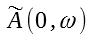of input pulses in such a way that GVD-induced degradation is eliminated, or at least reduced substantially. Clearly, if the spectral amplitude is modified asthe GVD will be compensated exactly, and the pulse will retain its shape at the fiber output. Although, it is not easy to implement this transformation, one can come close to it by prechirping optical pulses. For this reason, the prechirping technique attracted attention as early as 1988 and has been implemented in several experiments to increase the fiber-link lengh.

Prechirp Technique

The following figure can help us in understanding how prechirping helps.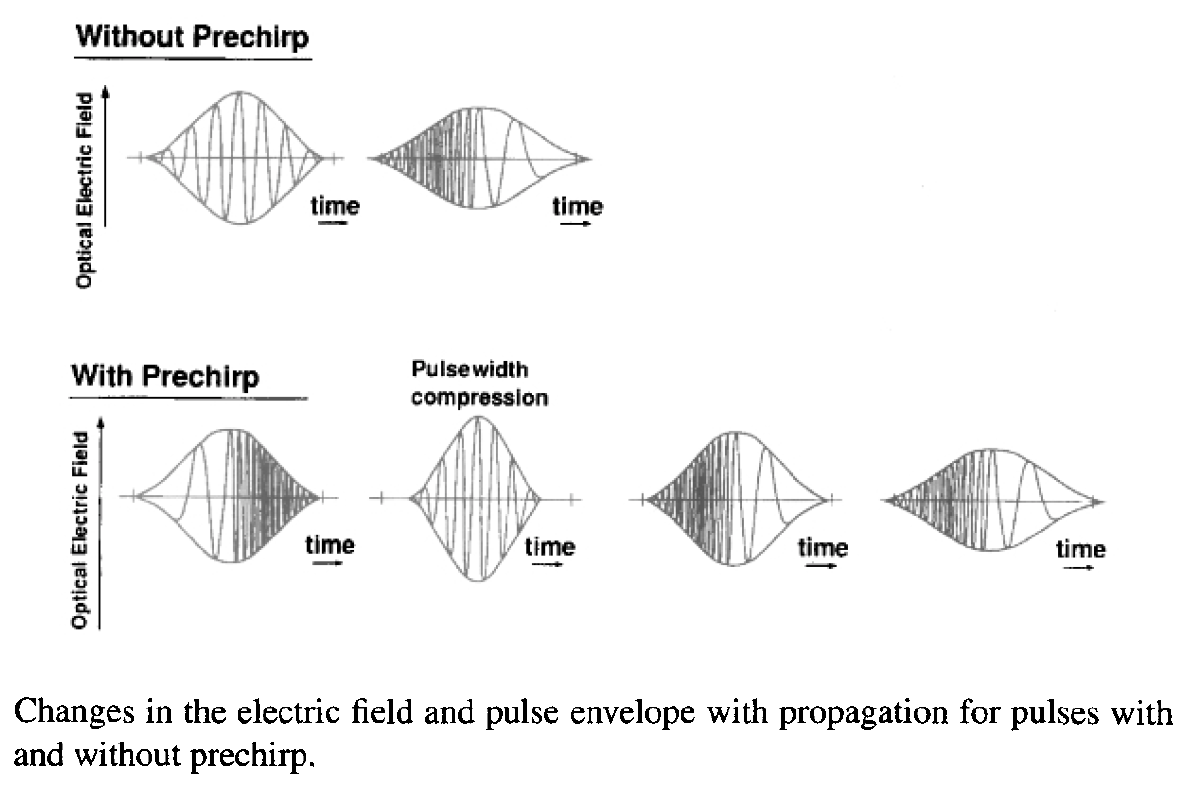Without prechirp, optical pulses spread monotonically because of chirping induced by dispersion. However, as discussed in the dispersion-induced pulse broadening tutorial and shown in the figure above, for values of C such that β2C < 0, a chirped pulse compresses initially before its width increases. For this reason, a suitably chirped pulse can propagate over longer distances before it broadens outside its allocated bit slot. As a rough estimate of the improvement, assume that pulse broadening by a factor of up to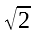is tolerable. The maximum transmission distance is found to be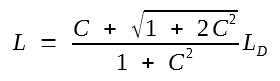where LD = T02/|β2| is the dispersion length. For unchirped Gaussian pulses, C = 0 and L = LD. However, L increases by 36% for C = 1. The maximum improvement by a factor ofoccurs for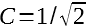. These features clearly illustrate that the prechirp technique requires careful optimization. Even though the pulse shaped is rarely Gaussian in practice, the prechirp technique can increase the transmission distance by 50% or more. As early as 1986, a super-Gaussian model predicted such an improvement.

In the case of direct modulation, the semiconductor laser chirps each optical pulse automatically through the carrier-induced index changes. Unfortunately, the chirp parameter C is negative for directly modulated semiconductor lasers. Since β2 in the 1.55-μm wavelength region is also negative for standard fibers, the condition β2C < 0 is not satisfied. In fact, the chirp induced by direct modulation reduces the transmission distance drastically when standard fibers are used. In contrast, if dispersion-shifted fibers with normal GVD (β2 > 0) are employed, the same chirp helps to improve the system performance. In deed, such fibers are routinely employed for metro networks to incorporate prechirping-induced dispersion compensation.

In the case of external modulation, optical pulses are nearly chirp-free. The prechirp technique in this case imposes a frequency chirp on each pulse with a positive value of the chirp parameter C so that the condition β2C < 0 is satisfied. In a simple approach, the carrier frequency of the DFB laser is first modulated (FM) before the laser output is passed to an external modulator for amplitude modulation (AM). The resulting optical signal exhibits simultaneous AM and FM. This technique falls in the category of electronic compensation because the FM of the optical carrier is realized by modulating the current injected into the DFB laser by a small amount (~1 mA). Although such a direction modulation of the DFB laser also modulates the optical power sinusoidally, the magnitude is small enough that it does not interfere with the detection process.

To see how FM of the optical carrier generates a signal that consists of chirped pulses, we assume for simplicity that the pulse shape is Gaussian. The optical signal can then be written in the form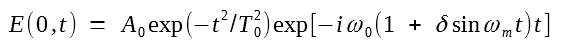where the carrier frequency ω0 of the pulse is modulated sinusoidally at the frequency ωm with a modulation depth δ. Near the pulse center, sin(ωmt) ≈ ωmt, and the equation above becomes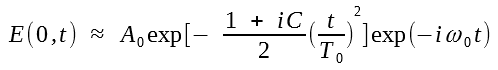where the chirp parameter C is given by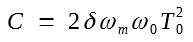Both the sign and magnitude of the chirp parameter C can be controlled by changing the FM parameters δ and ωm.

Phase modulation of the optical carrier also leads to a positive chirp, as can be verified by usingand using cos x ≈ 1 - x2/2. An advantage of the phase-modulation technique is that the external modulator itself can modulate the carrier phase. The simplest solution is to employ an external modulator whose refractive index can be changed electronically in such a way that it imposes a frequency chirp with C > 0. As early as 1991, a 5-Gb/s signal was transmitted over 256 km using a LiNbO3 modulator such that values  of C were in the range of 0.6 to 0.8. Other types of modulators, such as an electroabsorption modulator or a Mach-Zehnder modulator, can also chirp the optical pulse with C > 0, and have been used to demonstrate transmission beyond the dispersion limit. With the development of DFB lasers integrated with an electroabsorption modulator, the implementation of the prechirp technique became quite practical. In a 1996 experiment, a 10-Gb/s NRZ signal was transmitted over 100 km of standard fiber using such a transmitter. By 2005, link length of up to 250 km became possible through chirp management at the transmitter end.

Prechirping of an bit stream can also be accomplished through amplification of the optical signal. This technique, first demonstrated in 1989, amplifies the transmitter output using a semiconductor optical amplifier (SOA) operating in the gain-saturation regime. Physically speaking, gain saturation leads to time-dependent variations in the carrier density, which, in turn, chirp the amplified pulse through changes in the refractive index. The amount of chirp depends on the input pulse shape and is nearly linear over most of the pulse. The SOA not only amplifies the pulse but also chirps it such that the chirp parameter C > 0. Because of this chirp, the input pulse can be compressed in the fiber with β2 < 0. Such a compression was observed in an experiment in which 40-ps input pulses were compressed to 23 ps when they were propagated over 18 km of standard fiber.

The potential of this technique for dispersion compensation was demonstrated in a 1989 experiment by transmitting a 16-Gb/s signal over 70 km of fiber. In the absence of amplifier-induced chirp, the transmission distance at 16 Gb/s is limited to about 14 km for a fiber with D = 15 ps/(km-nm). The use of the amplifier in the gain-saturation regime increased the transmission distance five-fold. It has the added benefit that it can compensate for the coupling and insertion losses that invariably occur in a transmitter by amplifying the signal before it is launched into the optical fiber. Moreover, this technique can be used for the simultaneous compensation of fiber losses and GVD if SOAs are used as in-line amplifiers.

A nonlinear medium can also be used to prechirp the pulse. As discussed in the nonlinear phase modulation part of the nonlinear optical effects tutorial, the nonlinear phenomenon of self-phase modulation (SPM) chirps an optical pulse as it propagates down a fiber. Thus, a simple prechirp technique consists of passing the transmitter output through a fiber of suitable length before launching it into the communication link. The phase of the optical signal is modulated by SPM aswhere P(t) is the power of the pulse and Lm is the length of the nonlinear fiber. In the case of Gaussian pulses for which P(t) = P0exp(- t2/T02), the chirp is nearly linear, and the equation above can be approximated bywhere the chirp parameter is given by C = 2γLmP0. For γ > 0, the chirp parameter C is positive, and is thus suitable for dispersion compensation. The transmission fiber itself can be used for chirping the pulse. This approach was suggested in a 1986 study; it indicated the possibility of doubling the transmission distance by optimizing the average power of the input signal.

Novel Modulation Format

The dispersion problem can also be alleviated to some extent by adopting a suitable modulation format for the transmitted signal. In an interesting approach, referred to as dispersion-supported transmission, the frequency-shift keying (FSK) format was employed for signal transmission. The FSK signal is generated by switching the laser wavelength by a constant amount Δλ between 1 and 0 bits while leaving the power unchanged. During propagation inside the fiber, the two wavelengths travel at slightly different speeds. The time delay between the 1 and 0 bits is determined by the wavelength shift Δλ and is given by ΔT = DLΔλ. The wavelength shift Δλ is chosen such that ΔT = 1/B. The figure below shows schematically how the one-bit delay produces a three-level optical signal at the receiver. In essence, because of fiber dispersion, the FSK signal is converted into a signal whose amplitude is modulated. The signal can be decoded at the receiver by using an electrical integrator in combination with a decision circuit.Several transmission experiments have shown the usefulness of the dispersion-supported transmission scheme. All these experiments were concerned with increasing the transmission distance of a 1.55-μm lightwave system operating at 10 Gb/s or more over standard fibers exhibiting large GVD (about 17 ps/km/nm). In a 1994 experiment, transmission of a 10-Gb/s signal over 253 km of standard fiber was realized with this approach. By 1998, in a 40-Gb/s field trial, the siganl was transmitted over 86 km of standard fiber. Clearly, the transmission distance can be improved by a large factor by employing the FSK technique when the system is properly designed.

Another approach to increasing the transmission distance consists of employing a modulation format for which the signal bandwidth at at a given bit rate is smaller compared with that of the standard on-off keying. One scheme makes use of duobinary coding. This coding scheme reduces the signal bandwidth by 50% by adding two successive bits in the digital bit stream, thus forming a three-symbol duobinary code at half the bit rate. Since both the 01 and 10 combinations add to 1, the signal phase must be modified to distinguish between the two. Since the GVD-induced degradation depends on signal bandwidth, transmission distance is considerably larger for a duobinary signal.

In a 1994 experiment designed to compare the binary and duobinary schemes, a 10-Gb/s signal could be transmitted over distances 30 to 40 km longer by replacing binary coding with duobinary coding. The duobinary scheme can be combined with the prechirp technique. Indeed, transmission of a 10-Gb/s signal over 160 km of standard fiber was realized in 1994 by combining duobinary coding with an external modulator capable of producing a frequency chirp with C > 0. Since chirping increases the signal bandwidth, it is hard to understand why it would help. It appears that phase reversals occurring in practice when a duobinary signal is generated are primarily responsible for improvement realized with duobinary coding. Another dispersion-management scheme, called the phase-shaped binary transmission, has also been proposed to take advantage of phase reversals. The use of duobinary transmission increases signal-to-noise requirements and requires decoding at the receiver. Despite these shortcomings, it is useful for upgrading the existing terrestrial lightwave systems to bit rate of 10 Gb/s and more.

Digital Signal Processing

Considerable progress has been made in recent years to implement the transformation given in the equation below within the transmitter as accurately as possible. (refer to the beginning of this subsection)The basic idea is that this transformation is equivalent to a convolution in the time domain that can be carried out electronically using digital signal processing.

Figure (a) below shows the scheme proposed in 2005. It makes use of digital signal processing together with digital-to-analog conversion to determine the exact amplitude and phase of each bit and then generate the entire bit stream by applying the resulting electronic signal to a dual-drive Mach-Zehnder modulator.The time-domain convolution that corresponds to the transformation given in the equation above is calculated by using a look-up table of the incoming bit sequence stored in memory. The accuracy of the convolution depends on the number of consecutive bits employed for calculating it. Figure (b) above shows the numerically estimated eye opening penalty as a function of fiber length when 5, 9, and 13 consecutive bits are used for this purpose and compares it with the uncompensated case (dashed curve). In the uncompensated case, a penalty of 2 dB occurred at 80 km (accumulated dispersion da = 1360 ps/nm). With the 13-bit electronic precompensation, the link length could increased to close to 800 km (da = 13,600 ps/nm), indicating the dramatic improvement possible with such a scheme. In principle, any link length can be realized by increasing the number of consecutive bits employed for calculating the convolution more and more accurately. A field-programmable gate array was used for digital signal processing in a 2004 experiment.

In a different approach to this problem, GVD was precompensated using only intensity modulation of the optical signal. At a first sight, such an approach should fail because the transformation given in the above equation cannot be realized through pure intensity modulation. However, in the case of direct detection, the phase information at the receiver is discarded. One can thus use the phase at the receiver end as an additional degree of freedom. For a given optical power pattern at the receiver, it is possible to find the predistorted injection current required for direct modulation of a semiconductor laser that will provide that pattern, provided one knows the specific relation between the intensity and phase for that laser. In the 2009 experiment, an artificial neural network was used to find the injection current, which was then used to modulate directly a semiconductor laser. The resulting 10-Gb/s signal could be transmitted over 190-km of standard fiber (da = 3,500 ps/nm). Numerical simulations showed that dispersion precompensation over up to 350 km of fiber was possible with this technique.

#### 3. Dispersion Compensation at the Receiver

Electronic dispersion compensation within the receiver is most attractive because it requires only suitably designed integrated-circuit chips. With recent advances in the analog and digital signal processing, this approach has become realistic for modern lightwave systems. The main difficulty lies in the fact that electronic logic circuits must operate at a high speed close to the bit rate, or the symbol rate if more than one bit/symbol is transmitted using advanced modulation formats. Dispersion-equalizing circuits operating at bit rates of 10 Gb/s were realized in 2000, and by 2007 such circuits were being used for systems operating at 40 Gb/s.

Since direct detection recovers only the amplitude of the transmitted signal, no linear equalization technique can recover a signal that has spread outside its allocated bit slot. Nevertheless, several nonlinear signal processing techniques, developed originally for radio and cable networks, have been adopted for lightwave systems. Two commonly used ones are known as the feed-forward equalizer (FFE) and the decision-feedback equalizer (DFE), and both of them have been realized in the form of integrated-circuit chips operating at bit rates of up to 40 Gb/s. The figure below shows a design in which the two equalizers are combined in series.A feed-forward equalizer consists of a transversal filter in which the incoming electronic signal x(t) is split into a number of branches using multiple tapped delay lines and their outputs are then combined back to obtainwhere N is the total number of taps, Tc is the delay time (about 50% of the bit slot), and cm is the relative weight of mth tap. Tap weights are adjusted in a dynamic fashion using a control algorithm such that the receiver performance is improved. The error signal for the control electronics may correspond to maximization of the "eye opening" or of the Q factor provided by an eye monitor within the receiver.

A decision-feedback equalizer, as its name suggest, makes use of the feedback provided by a decision circuit. More precisely, a fraction of the voltage at the output of the decision circuit is subtracted from the incoming signal. Often, such a circuit is combined with a feed-forward equalizer, as shown in the figure above, to improve the overall performance. Although digital signal processing (DSP) can be employed for both equalizers, such electronic circuits are realized in practice using analog signal processing because they consume less power. An advantage of such circuits is that they can also compensate for PMD simultaneously.

Another electronic equalizer, known as the maximum likelihood signal estimator (MLSE), is based on digital signal processing and thus requires an analog-to-digital converter after the photodetector. It makes use of the Viterbi algorithm, conceived in 1967 and used widely in cellular networks. This algorithm works by examining multiple bits simultaneously and finding the most likely bit sequence for them. As it is not based on a specific form of distortion, an MLSE equalizer can compensate for both the GVD and PMD simultaneously.

A 2007 study was devoted to understand to what extent different electronic equalizers improve the performance of 10.7 Gb/s systems, making use of on-off keying with the RZ or the NRZ format, when they are affected by the GVD and PMD individually or simultaneously. The figure below shows the measured optical SNR penalty as a function of fiber length [D = 17 ps/(km-nm)] when the signal was affected only by GVD (negligible PMD along the link). Several points are noteworthy. First, the penalty is considerably smaller for the NRZ format compared with the RZ format in all cases. This is understood by recalling that optical pulses are wider (or signal bandwidth is smaller) in the case of the NRZ format. Second, the signal can be transmitted over longer distances when an electronic equalizer is employed. Assuming that at most a 2-dB penalty can be tolerated, the distance is 54% and 43% longer in the case of the NRZ and RZ formats, respectively, when the combination of FFE and DFE is employed. Third, the MLSE equalizer works better in both cases. In the case of the NRZ format, the fiber length increases from 50 to 110 km at the 2-dB penalty point.The results for PMD compensation showed that the RZ format is more tolerant of the PMD than the NRZ format. The use of electronic equalizers improved considerably the PMD level that could be tolerated, and the most improved occurred again for the MLSE equalizer. However, when both the GVD and PMD were present simultaneously, the tolerable PMD level was comparable for the RZ and NRZ formats.

Electronic compensation of dispersion can be carried out much more readily if both the signal amplitude and phase are detected at the receiver. Moreover, the compensation of PMD requires that this information be available for both polarization components of the received optical signal. The use of coherent detection makes this possible, and several experiments have implemented this approach in recent years.

The figure below shows a coherent receiver in which the use of phase and polarization diversity with four photodiodes permits the recovery of the amplitude and phases for both polarization components. A polarization beam splitter splits the incoming signal into its orthogonally polarization components, Ex and Ey, where are then combined with the output of a local oscillator using two 3 x 3 couplers acting as 90° hybrids. The four photodiodes recover the real and imaginary parts of ExElo* and EyElo*, respectively, from which both the amplitude and phases can be obtained. The local oscillator converts the optical signal to the microwave domain while keeping its amplitude and phase intact.Compensation of GVD is easily implemented in the frequency domain using an all-pass filter whose transfer function is that inverse of that given in the equation below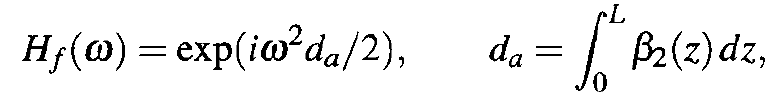This step requires digitization of the complex field, computation of its numerical Fourier transform, multiplication by H(ω), and then inverse Fourier transform of the resulting digital signal. All these steps can be implemented with digital signal processing.

GVD can also be compensated in the time domain by converting the transfer function in the equation above to an impulse response by taking its Fourier transform:It is not easy to implement this impulse response digitally because its infinite duration makes it noncausal. However, if the impulse response is truncated appropriately, it can be implemented using a finite-impulse-response filter with a tapped delay line. The required number of taps depends both on the symbol rate and da; it exceeds 200 for a 10 Gbaud signal transmitted over 4,000 km of optical fiber.

Compensation of PMD can be carried out in the time domain using the inverse of the Jones matrix that corresponds to propagation of optical signal through the fiber link. However, it is not easy to find this matrix. Moreover, the effects of PMD change in a dynamic fashion, indicating that this matrix also changes with time. In the case of modulation formats such as DPSK and QPSK, one solution is to construct the inverse matrix from the received signal itself using an algorithm known as the constant modulus algorithm. Such an algorithm was used with success in a 2007 experiment in which a 42.8-Gb/s signal, modulated using the so-called dual-polarization QPSK (DP-QPSK) format was transmitted over 6400 km at a symbol rate of 10.7 Gbaud.

In the case of differential formats such as DPSK, the phase of the optical signal at the receiver can also be recovered without using a local oscillator by a technique known as self-coherent. In this scheme, the use of a Mach-Zehnder interferometer with one-bit delay between its two arms allows phase recovery. The same scheme can be employed even for traditional RZ and NRZ systems (making use of on-off keying) to recover the optical phase at the receiver and use it to construct the full optical field. The figure below shows how two photodetectors after the Mach-Zehnder interferometer can be used to reconstruct the field and use it for dispersion compensation with suitable electrical processing. This technique was employed in a 2009 experiment in which a 10-Gb/s signal could be transmitted over nearly 500 km of standard fiber in spite of more than 8,000 ps/nm of dispersion accumulated over the fiber link. Numerical simulations indicated that dispersion over more than 2000 km of fiber link can be compensated with this approach.Digital Backpropagation

Knowledge of the full optical field at the receiver permits another approach that can compensate not only for the dispersive effects but also for all kinds of nonlinear effects that degrade the signal during its transmission through the fiber link. This approach is known as digital backpropagation and is based on a simple idea: numerical back-propagation of the received signal, implemented with digital signal processing, should recover fully the original optical field at the transmitter end if all fiber-link parameters are known. This idea is attracting attention in recent years because of its potential for compensating for all degradations simultaneously.

It is not easy to implement backpropagation of the received signal digitally in real time because of the speed limit of current electronics. In practice, each WDM channel is translated to the baseband (without the optical carrier) using coherent detection, resulting in a complex signal Ek = Akexp(iφk) for the kth channel. The analog-to-digital converter should sample this field with sufficient temporal resolution. The number of sample points per symbol is relatively small (2 to 4) with the current state of digital signal processing, and one must adopt up-sampling to ensure sufficient temporal resolution. However, it is not possible to process the entire time-domain signal simultaneously. A parallel scheme is typically employed using a finite-impulse-response filter, instead of the conventional Fourier transform technique. This scheme was used in a 2008 experiment in which three 6-Gbaud WDM channels were transmitted over 760 km using the binary PSK format and was found to perform better than two other dispersion-compensation techniques.

The compensation of polarization-multiplexed WDM channels is more complicated because it requires the recovery of both polarization components of the optical signal for each channel and their digital backpropagation by solving two coupled NLS equations. In a 2009 experiment, a detection scheme similar to that of the previous figure, was employed to recover the amplitudes and phases of the two polarization components after three 6-Gbaud WDM channels were transmitted over 1440 km of using a 80-km-long recirculating fiber loop. The digitized complex amplitudes were backpropagated with the split-step Fourier method. The Q factor of the central channel after backpropagation depended on the step size. It increased from a low value of 4.5 dB to near 14 dB with a relatively large step size of 20 km. These results show that digital back-propagation is likely to become a practical technique with continuing improvements in the speed of electronics.